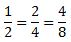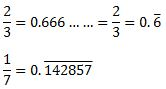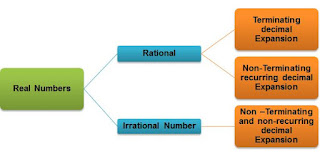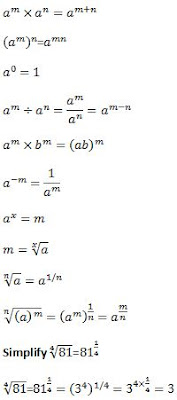## Revision Notes of Ch 1 Number Systems Class 9th Math

Topics in the Chapter

• Number System
• Types of Real Numbers
• Equivalent Rational Number
• Finding a rational Number between Two Rational Numbers
• Finding n rational numbers between Two Rational Numbers.
• Finding n rational numbers between two Rational Numbers.
• Locating Irrational Numbers on Number Line
• Decimal Expansion of Real Numbers
• Representing Recurring Decimal Expansion
• Representing √x Geometrically
• Finding Irrational Numbers between two rational numbers.
• Rationalisation
• Laws of Exponent

Number System

Real Numbers

→ The numbers which exist are known as rational numbers.

→ All real numbers can be represented on Number line.

→ Every real number is represented by a unique point on the number line. Also, every point on the number line represents a unique real number.

Types of Real Numbers

Rational Numbers

→ The numbers in the form of p/q where p and q integers and q≠0 are known as rational numbers.
Examples: -2/3, 4/5, -7, 3/2

Irrational Numbers

→ Real Numbers which cannot be expressed as ratio of two integers.
Examples: π, √3, √5

Equivalent Rational Number

→ All whole numbers, natural numbers, integers are rational numbers.

→ Equivalent Rational Numbers are rational number cannot have unique representation as p/q where p and q are integers and q≠0.

→ For example 1/2 can also be written as→ Rational number in simplest form is represented in the form of p/q where p and q integers and q≠0 also, p and q are co-prime numbers.

→ 1/2 is simplest form of 2/4, 4/8 as 1 and 2 are co-prime numbers.

Other examples: 3/4=6/10=9/12 is set of equivalent rational numbers. In ¾ , 3 and 4 are co-prime Numbers. ¾ is rational number in simplest form.

Finding a rational Number between Two Rational Numbers

• To find a rational number between two rational numbers a and b

→ Find d = (a+b)/2

→ d is the rational number between two rational numbers.

Question: Find a rational number between 3/5 and 4/5.

Solution

d=(3/5+4/5)/2 = (7/5)/2 = 7/10

• Note: There can be infinite rational numbers between two rational numbers.

Finding n rational numbers between Two Rational Numbers.

→ Find d = (b-a)/(n+1)

→ Then the n numbers between a and b are a+d, a+2d,….a+(n-1)d, a+nd.

→ Find all these values.

Question. Find 4 rational numbers between 5 and 5½.

We have to find 4 rational numbers between 5 and 5½ i.e between 5 and 11/2.
Here, n=4 as we have to find 4 rational numbers between a=5 and b=11/2
Find d = (b-a)/(n+1)
Here,
d=(11/2-5)/(4+1)=1/10

So, the four rational numbers are
a+d=5+1/10=51/10
⇒ a+2d=52/10
⇒ a+3d=53/10
⇒ a+4d=54/10

Therefore, the required 4 numbers are 51/10, 52/10, 53/10, 54/10.
In simpler form: 51/10, 26/5, 53/10, 27/5

Finding n rational numbers between two Rational Numbers.

(i) Find d = (b-a)/(n+1)
(ii) Then the n numbers between a and b are a+d, a+2d,….a+(n-1)d, a+nd.
(iii) Find all these values.

We have to find 4 rational numbers between 5 and 5½ i.e between 5 and 11/2.
Here, n=4 as we have to find 4 rational numbers between a=5 and b=11/2
Find d = (b-a)/(n+1)
Here,
d=(11/2 - 5)/(4+1)=1/10
So, the four rational numbers are
a+d=5+1/10=51/10
⇒ a+2d =52/10
⇒ a+3d =53/10
⇒ a+4d =54/10

Therefore, the required 4 numbers are 51/10, 52/10, 53/10, 54/10.
In simpler form: 51/10, 26/5, 53/10, 27/5

Locating Irrational Numbers on Number Line

→ Any real number is called irrational, if it cannot be written in the form p/q, where p and q are integers and q ≠ 0.
Examples: π, √2,√3

Question: Locating √2 in Number Line.(i) On Number line, marks point O which is 0, and 1 is marked as A.

(ii) Now a construction is done AB perpendicular to OA, that is number line. AB is equal to OA. Both equal to 1 unit. Since, OA represent 1 on number line.

(iii) Point O is joined by Point B. So, we have right angled triangle, OAB, right angled at A.

(iv) By applying Pythagoras theorem, we getQuestion: Locate √3 on Number Line→ The process is similar to previous case. We have to take base as OP=√2, and from C draw a line perpendicular CD equal to one Unit. By Pythagoras Theorem, we get OD= √3,

Note: In similar way we can fine √n on number line if √(n-1) .

Decimal Expansion of Real Numbers

→ Decimal expansion of any number can be obtained by performing Long division by dividing numerator by denominator.
Example: Decimal Expansion of 2/3 =0.66666……. (6 repeating)Terminating Decimal Expansion

→ The decimal expansion terminates or ends after a finite number of steps.
Examples: 9/8=1.125

Recurring Decimal Expansion

→ The decimal Expansion do not terminates but a pattern of digits repeats in the decimal expansion.
Examples: 2/3=0.666……
1/7=0.142857142857142857 here 142857 is repeated.

Representing Recurring Decimal Expansion

→ It Can be represented by putting bar over repeating terms.Non-Terminating Non-recurring Decimal Expansion

→ The decimal expansion, neither repeats, not terminates.
• Examples
π=3.14596…………….
√2=1.414…………….
2.3030030003………

Note: Rational numbers will have either terminating or Non-terminating recurring decimal expansion.

→ Irrational numbers will always have non-terminating and non-recurring decimal expansion.Converting Terminating Decimal Number into p/q formRepresenting Recurring Decimal ExpansionSteps:

Step 1: Expanding 4-5 area on number line in ten parts.

Step 2: Divide it in 10 parts. Each part is 4.1, 4.2, 4.3, 4.4,4,5,4.6, 4.7.4.8, 4.9

Step 3: Now divide 4.2 to 4.3 part in 10 parts. Each part will be 4. 21, 4,22, 4.23, 4.24. 4.25. 4.26, 4.27, 4.28, 4.29….

Step 4: Again magnifying and dividing 4.261, 4.262, 4.263, 4.264, 4.26,4.27, 4.28. 4.29..

Step 5: 4.26 to 4.27 is divided into 10 parts. We will get 4.26, the point is 4.262626…so, it is just more than 4.2626..

Representing √x GeometricallyStep 1: On a Line we draw AB= x

Step 2: Extend AB to C. Such that BC=1. AC=x+1

Step 3: Take mid-point of AB as O. With OC as arc draw a semi circle.

Step 4: Draw BD perpendicular to AC. BD represents √x . Why this so, you will understand if you go through the proof.

Proof:

OD = OC = (x+1)/2 Radius of Same Arc

From diagram:Finding Irrational Numbers between two rational numbers.

Step 2: First write rational numbers in decimal form.

Step 2:  Write three such decimal numbers between thoese decimal numbers which are having non-terminating and non-repeating decimal expnansion.

Q. Find three Irrational Numbers between 3/5 and 4/5.
3/5 = 0.6
4/5 =0.8

The three irrational numbers between 0.6 and 0.8 can be
0.61611611161111611111...............
0.67677677767777677777.............
0.707007000700007000007......................

Rationalisation

⟶ It is the Process of making denominator rational number if it is irrational. It is multiplied by same number in denominator and numerator.Laws of Exponent